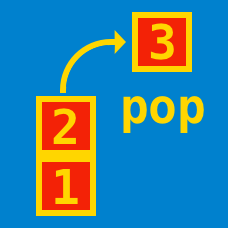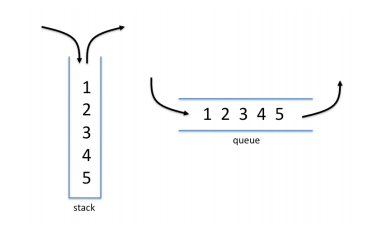Computer Science

# Abstract Data Types: Level 1-2 Challenges

In a stack $s$, TIM is equivalent to s.push(s.pop() * s.pop()) and SUM is equivalent to s.push(s.pop() + s.pop()). What will the following stack machine evaluate the operations as?

 1 2 3 4 5 6 7 8 9 PUSH 1 PUSH 2 PUSH 3 PUSH 4 PUSH 5 SUM TIM SUM TIMWhich line of this implementation of a queue should be changed in order to obtain a stack?

## C++

  1 2 3 4 5 6 7 8 9 10 11 12 13 14 15 16 17 18 19 20 21 22 23 24 25 26 27 28 29 30 31 #include struct thing { int size; /* size of contents */ int bottom; /* first used location */ int top; /* first unused location */ int *elements; /* array of elements */ }; struct thing *thingCreate(int size) { struct thing *t; t = malloc(sizeof(*t)); t->size = size; t->bottom = t->top = 0; t->elements = malloc(sizeof(int) * size); return t; } void Push(struct thing *t, int value) { t->elements[t->top++] = value; } int Pop(struct thing *t) { return t->elements[t->bottom++]; } 

Consider the following algebraic data type in Haskell:

 1 data X a = Nil | Node a (X a) (X a) 

What is the type of data strucutre that X entails?

Which of the following sets of data would be the best fit to be stored in an associative array?How many stacks are required to implement a queue if no other data structures such as arrays or linked lists are available?

×